# How to Calculate Real Eigenvalues of a Matrix of Arbitrary Dimension Numerically: A Tutorial on Numerical Methods with a C Program

Knoji reviews products and up-and-coming brands we think you'll love. In certain cases, we may receive a commission from brands mentioned in our guides. Learn more.
Calculation of real eigenvalues of a square matrix is often required in physical problems. In most of the cases it is not possible to find the eigenvalues by hand and we need to use numerical methods. In this article I have discussed one such method with

Every square matrix has eigenvalues whose total number is equal to the dimension of the square matrix. This is because the eigenvalues are the solutions of an equation called secular equation or characteristic equation whose degree is same as the dimension of the given matrix. In general the eigenvalues may be real or complex, distinct or degenerate. But in many physical problems it is often required to calculate the real eigenvalues of a matrix. There are very few cases when the eigenvalues can be calculated by hand, and thus most of the cases we need to rely upon the numerical methods. In this article I am going to discuss how to numerically determine real eigenvalues of a square matrix of arbitrary dimension.

The Method

Let us label the square matrix under consideration by “A” and its eigenvalues by “x”. Then our method of finding eigenvalues involves the following three steps.

1. Subtract from A, x times an identity matrix having the same dimension as A.
2. Determine the secular equation which is basically same as finding determinant of the modified matrix.
3. Find the roots of the secular equation.

I will discuss these steps with a computer code written in C language.

First Step: Subtract x*Identity from A

At first we need to provide all the elements of the matrix and its dimension. See the first part of the program below.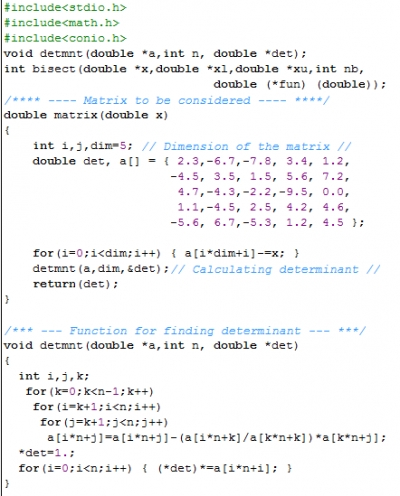Here, for example, we have taken a 5 by 5 matrix. The matrix elements are stored in the array a[]. In principle we should take a two dimensional matrix of dimension 5 by 5, but since we are going to pass the array to a function, for ease of array handling we have taken a one dimensional array of length 5*5=25.The subtraction of identity matrix of dimension 5 is obtained by subtracting x from each diagonal elements of A.

Second Step: Find the Determinant

To obtain the characteristic equation we need to find the determinant of the modified matrix. The method of normal expansion requires a lot of calculation and is slow also; so I have calculated the determinant using the method of Gaussian elimination, which is written in the function “detmnt”. After performing Gaussian elimination a matrix transforms to an upper triangular matrix and then the determinant simply becomes the product of the diagonal terms.

Third Step: Find the Roots of the Secular Equation

This is the most important part of the whole method. There are several methods to solve a polynomial equation but here I have chosen the method of bisection which is very effective for finding real roots though its rate of convergence is not very high. Bisection is applied whenever a bracketing pair of points is obtained in the search interval. See the code below for clarification.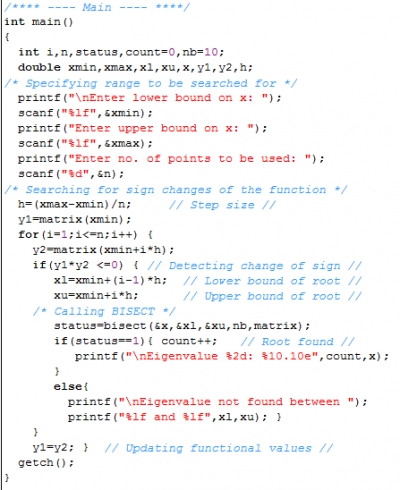The Method of Bisection

A bracketing pair requires that the functional values at those two points have opposite sign which in mathematical form becomes f(x1)*f(x2) = y1*y2<0. Whenever this condition is satisfied the pair of points is passed to a function called “bisect” in which the bracketing interval is bisected again and again ultimately to reach the actual root. One drawback of this method is that it will count multiple roots (roots with multiplicity greater than 1) as a single. The function is given below.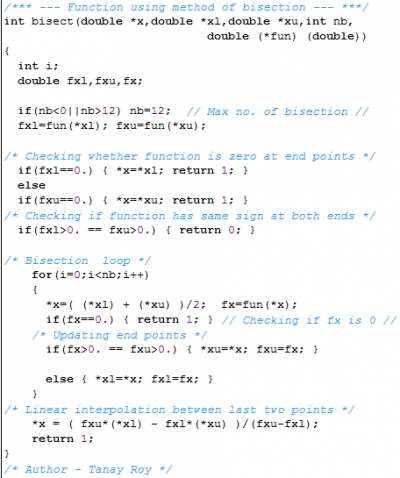How to Run the Program

At first copy all the three parts shown above in a single file and then provide your matrix elements and dimension appropriately. When you will run the program it will ask the range to be searched for and number points(n) to be used in that interval. Here you need to choose these inputs wisely to get all the real eigenvalues, i.e. you should have a rough of estimate of what might be the order of magnitude of the roots. Alternatively you can initially search a large range with less number of points, i.e. with large step size; then after getting the idea of the roots you can perform a fine search with smaller intervals and large number of points.

The matrix chosen here has three real and two imaginary eigenvalues. A typical input is shown below.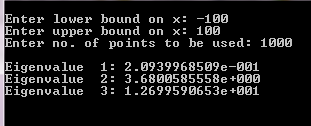In any case better result will be obtained if you use large number points but then the program will take longer time to give the answer.

This is how we can calculate real eigenvalues of a real matrix of arbitrary dimension.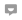Rashi Mehra
0
Posted on Oct 30, 2013Eunice Pacheco
0
Posted on Dec 13, 2012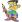roytanay
0
Posted on May 9, 2012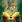deepa venkitesh
0
Posted on May 8, 2012